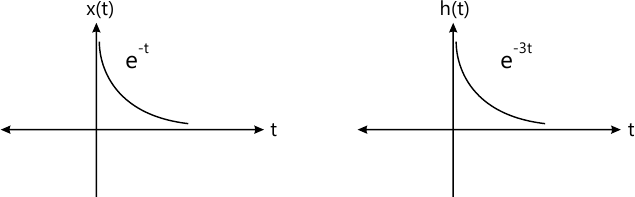MORE IN Signals & Systems
Total marks: --
Total time: --
INSTRUCTIONS
(1) Assume appropriate data and state your reasons
(2) Marks are given to the right of every question
(3) Draw neat diagrams wherever necessary

Answer any one question from Q1 and Q2
1 (a) A discrete time signal is given below. Check for following system properties:
i) Static/Dynamic
ii) Linearity
iii) Causality
iv) Stability
y(n)=8 cos x(n)
6 M
1 (b) Sate and prove the convolution Integral property. With suitable block diagram & mathematical equation.
3 M
1 (c) Find Y(n) discrete time signal convolution Integral.
x(n)= (u(n) -u (n-4)) and h(n)= {1,1,1,1}
3 M

2 (a) Find given signal is whether energy signal or power signal. Find its value x(t)=rect (t); for t=-1 to t+1
3 M
2 (b) Find the convolution of flowing signal plot Y(t).6 M
2 (c) Find Even and Odd Component for given signal.
x(t)=1+3t+4 sin (t) + 6 cos (3t)
3 M

Answer any one question from Q3 and Q4
3 (a) State and Prove the following property of Laplace Transform.
i) Periodic Signal Property
ii) Time Scaling Property
6 M
3 (b) Find the Quadrature Fourier series for the full wave rectifier output signal. With amplitude 'A' and periodic 0 to π.
6 M

4 (a) Find the Initial Value and Final Value of the signal x(t) its Laplace Transform $X(S) = \dfrac {2S+3} {S^2 +5S -7}$
6 M
4 (b) Show that Rectangular function in time domain to become Sinc function in Frequency Domain.
6 M

Answer any one question from Q5 and Q6
5 (a) Determine the Auto Correlation Function Energy Spectral density of x(t)=cos πt [u(t+2)] -u [t-2] and sketch the auto-correlation.
10 M
5 (b) State the properties of Energy Spectral Density, Power Spectral Density, Autocorrelation and Cross Correlation.
8 M
5 (c) Explain the properties of Probability, CDF and PDF.
8 M

6 (a) The probability density function of a random Variable 'x' is defined as  Find
i) Constant ii) P(1
10 M
6 (b) Draw and explain the following probability distribution model.
i) Gaussian distribution Model.
ii) Uniform Distribution Function.
8 M
6 (c) Find Cross-Correlation of following discrete time signal.
x(n)={1,2,3,4} and y(n)={3,2,1,0}
8 M

More question papers from Signals & Systems